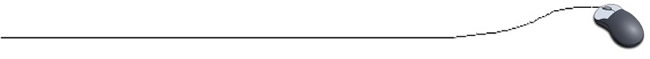Parallel Arrays
 The following arrays represent the data from a dog show.  Notice that the arrays do not all contain the same "type" of data.  It is often necessary to represent data in a "table" form, as shown below.  Such data, can be stored using parallel arrays.  Parallel arrays are several arrays with the same number of elements that work in tandem to organize data.dogname

round1

 18 22 12 17 15

round2

 20 25 16 18 17

**The true beauty of parallel arrays, is that each array
may be of a different data type.

In the data represented above, the first array is the dog's name, the second array is the dog's score in round 1 of the competition, and the third array is the dog's score in round 2 of the competition.  The arrays are parallel, in that the dog in the first element of the first array has the scores represented in the first elements of the second and third arrays.

String[ ] dogname = {"Wally", "Skeeter", "Corky", "Jessie", "Sadie"};
int[ ] round1 = {18,22,12,17,15};
int[ ] round2 = {20,25,16,18,17};

//Printing the dog competition information:

for(int index = 0; index < dogname.length; index++)
{
System.out.print(dogname[index] + " ");
System.out.print(round1[index] + " ");
System.out.println(round2[index]);
}